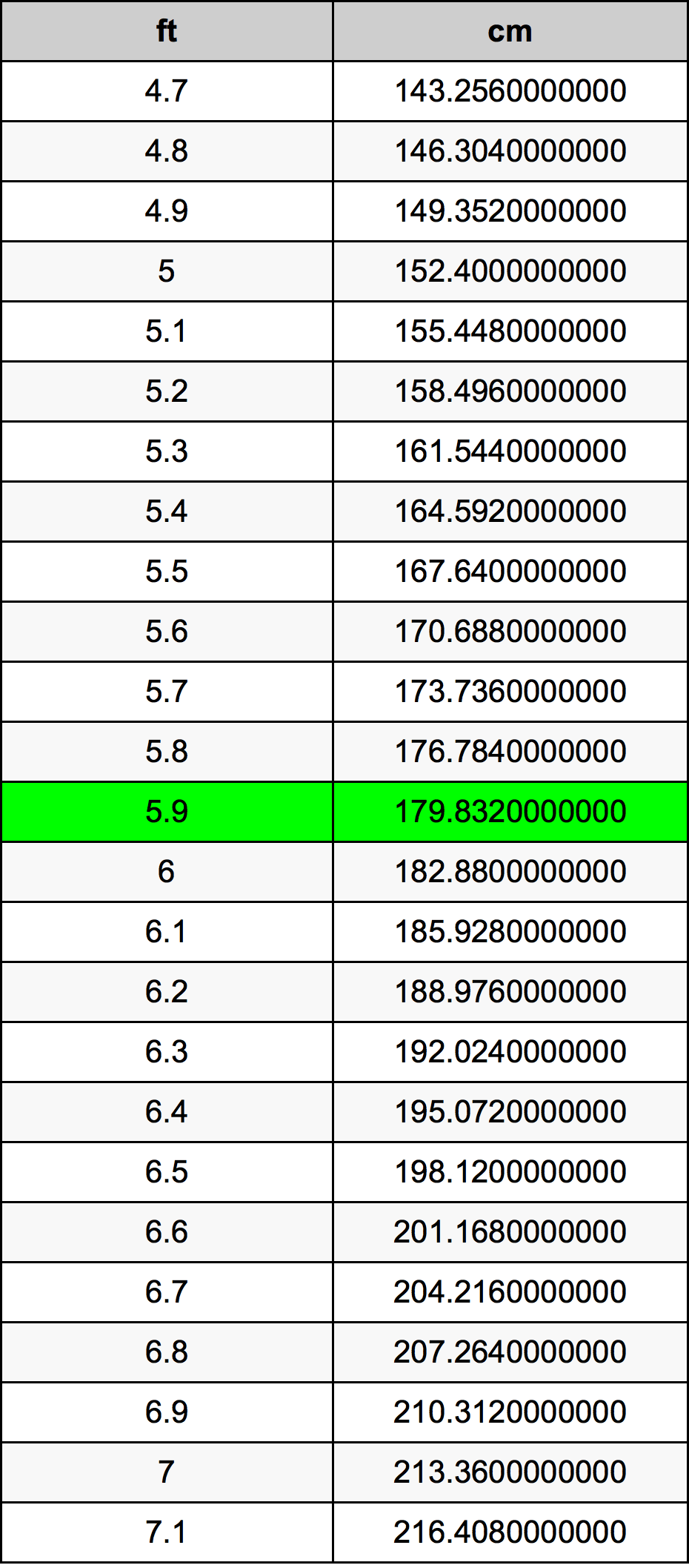Feet To Cm

# 5.9 ft to cm5.9 Feet to Centimeters

ft
=
cm

## How to convert 5.9 feet to centimeters?

 5.9 ft * 30.48 cm = 179.832 cm 1 ft
A common question is How many foot in 5.9 centimeter? And the answer is 0.1935695538 ft in 5.9 cm. Likewise the question how many centimeter in 5.9 foot has the answer of 179.832 cm in 5.9 ft.

## How much are 5.9 feet in centimeters?

5.9 feet equal 179.832 centimeters (5.9ft = 179.832cm). Converting 5.9 ft to cm is easy. Simply use our calculator above, or apply the formula to change the length 5.9 ft to cm.

## Convert 5.9 ft to common lengths

UnitLength
Nanometer1798320000.0 nm
Micrometer1798320.0 µm
Millimeter1798.32 mm
Centimeter179.832 cm
Inch70.8 in
Foot5.9 ft
Yard1.9666666667 yd
Meter1.79832 m
Kilometer0.00179832 km
Mile0.0011174242 mi
Nautical mile0.0009710151 nmi

## What is 5.9 feet in cm?

To convert 5.9 ft to cm multiply the length in feet by 30.48. The 5.9 ft in cm formula is [cm] = 5.9 * 30.48. Thus, for 5.9 feet in centimeter we get 179.832 cm.

## 5.9 Foot Conversion Table## Alternative spelling

5.9 Foot to cm, 5.9 Foot in cm, 5.9 ft to Centimeters, 5.9 ft in Centimeters, 5.9 Feet to cm, 5.9 Feet in cm, 5.9 ft to Centimeter, 5.9 ft in Centimeter, 5.9 Feet to Centimeter, 5.9 Feet in Centimeter, 5.9 Foot to Centimeter, 5.9 Foot in Centimeter, 5.9 Feet to Centimeters, 5.9 Feet in Centimeters